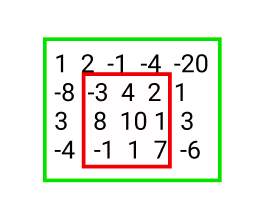Max Submatrix

Posted: 4 Mar, 2021
Difficulty: Moderate

PROBLEM STATEMENT

For example: For the ‘MAT’ given below, the maximum sum submatrix having a sum of 29 is highlighted in red.Input Format :
The first line of input contains an integer ‘T’ denoting the number of test cases. Then each test case follows.

The first line of each test case contains two space-separated integers ‘N’ and ‘M’ representing the number of rows and columns respectively. size of the matrix ‘MAT’.

The next ‘N’ lines of each test case contain ‘M’ single space-separated integers denoting the values of ‘MAT’.
Output Format :
For each test case, print the maximum sum over all submatrices in ‘MAT’.

Print the output of each test case in a separate line.
Note :
You are not required to print the expected output; it has already been taken care of. Just implement the function.
Constraints :
1 <= ‘T’ <= 100
1 <= ‘N’, ‘M’<= 100
-100000 <= ‘MAT[i][j]’ <=100000

Where 'MAT[i][j]' represents the value at cell(i, j).

Time limit: 1 secApproach 1

The brute force approach is we check for every possible submatrix in the given ‘MAT’.

• 4 nested loops are required to select every submatrix of the matrix.
• 2 nested loops are required to take the sum of all the elements of the submatrix.

Here is the algorithm :

1. We declare a variable ‘MAX_SUM’ in which we store the maximum sum over all submatrices in the matrix.
2. We run a loop for ‘i’ = 0 to ‘N’:
• We run a loop for ‘j’ = 0 to ‘M’:
• We run a loop for ‘k’ = ‘i’ to ‘N’:
• We run a loop for ‘l’ = ‘j’ to ‘M’:
• We declare a variable ‘tmpSum’ in which we store the sum of the current submatrix
• We run a loop for ‘q’ = ‘i’ to ‘k’:
• We run a loop for ‘r’ = ‘j’ to ‘l’:
• ‘tmpSum’ += mat[q][r]
• ‘maxSum’ = max(‘maxSum’, ‘tmpSum’)
3. Finally, we return ‘MAX_SUM’.